★ APPLICATIONS ★ DIVERS ★ GAUSS|Amstrad Action) ★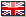Gauss Applications Divers
##### ★ Ce texte vous est présenté dans sa version originale ★  ★ This text is presented to you in its original version ★  ★ Este texto se presenta en su versión original ★  ★ Dieser Text wird in seiner Originalfassung präsentiert ★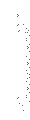\$( "#bt1" ).click(function() {\$( "#bc1" ).toggle( "slow", function(){var isHidden = \$(this).is(":hidden");});});With exam time rapidly approaching, many of you will fmd this computerised method for solving simultaneous equations very useful Any number of unknowns may be found in this faithful reproduction of the Gauss-Jordan elimination method Stuart McColl from Easthall in Glasgow is the man to thank.The routine will easily solve2x1 + 4x2 + 6x3 = 184x1 + 5x2 + 6x3 = 243x1 + x2 - 2x3 = 4When run, Gauss will prompt you for the number of equations you wish to solve (for the above this would be 3) Next you are asked for the number of variables (again, from the example, this would be 3). Note that the program only uses positional coefficients - it doesn't matter whether the equanon reads 2x1 + 4x2 + 6x3 = 18 or 2x + 4y + 6z = 18; the only input required is 2, 4, 6 and 8 (separated by a tap of the return key)Gauss works by implementing elementary row operations1 Reduce coefficient of x1 to 1 in equation one2 Eliminate x1 from all other equations by multiplying equation one and adding it to the others3 Repeat this for x2 , x3 and so onIf the resultant matrix is the identity matrix , 1 , the system has a unique solution The result for the earlier example would be printed as1 0 0 40 1 0 -20 0 1 3The first three columns in this example have indeed been reduced to the identity matrix for a three by three system This signifies that a unique solution exists Each row is a reduced equation which is equivalent to the initial equations The results are read asx1 + 00 + 00 = 400 + x2 + 00 = -200 + 00 + x3 = 3If no unique solution exists the results may be of the form:x1 + 2x2 + 00 = 11000 + x2 + x3 = 90This can be rearranged to give one variable in the shape of another The solutions to this example are x1 - 110 - 2x2 , x2 = 90 - x3, x3.AA#31

 ★ PUBLISHER: Amstrad Action★ YEAR: 1988★ AUTHOR: Stuart McColl (Easthall in Glasgow)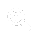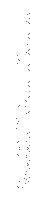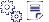Type-in/Listing:» Gauss    (Amstrad  Action)    LISTING    ENGLISHDATE: 2018-08-04DL: 10 foisTYPE: textSIZE: 3KoNOTE: Uploaded by CPCLOV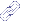Lien(s):» Coding Src's » Pointers - Windows System» Coding Src's » Graphic - Scroll - Bremse» Coding Src's » Sound - Sarabande» Coding Src's » Graphic - Circle Writer (Amstrad Computer User)» Coding Src's » Rotating Sphere» Coding Src's » Rocky 2 (Amstrad Action)